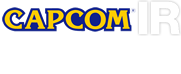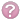#Font Size

••##(as of September 10, 2021)

These tables and graphs present our ROE, ROA and other financial data for the previous years. In recent years, we have increased our investments aimed at sustaining growth. This is why R&D expenditures and capital expenditures have been climbing. Furthermore, we are taking actions aimed at improving our financial soundness. One way is by reducing debt. We are also operating more efficiently, such as by reducing assets to raise the ROA and the turnover ratio for total assets.

### Financial IndexFinancial Index (historical data) (Excel: 32KB)

Click on the articles to see the descriptions of the indexes.

2017/3 2018/3 2019/3 2020/3 2021/3
R&D investment costs (Millions of yen) 27,720 29,077 27,038 25,843 25,375
Capital investment
(Tangible fixed assets, net of accumulated depreciation)

(Millions of yen)
2,622 2,918 2,439 2,504 2,570
Depreciation and amortization (Millions of yen) 5,980 4,706 3,228 2,795 2,791
Interest-bearing debt (Millions of yen) 16,111 9,788 8,315 6,735 5,606
Return on equity (ROE)（%） 11.6 13.4 14.4 16.9 22.6
Return on total assets (ROA)（%） 7.7 8.9 10.1 12.0 16.2
Net worth ratio（%） 65.4 68.4 71.9 69.5 73.8
Creditor's equity to total assets（%） 34.6 31.6 28.1 30.5 26.2
Current ratio（%） 285.0 354.0 391.2 303.5 390.9
Quick ratio (Acid-test ratio)（%） 149.1 220.0 288.5 227.6 295.6
Cash equivalent ratio（%） 81.8 172.1 228.3 183.1 218.6
Fixed ratio（%） 43.0 35.0 36.7 34.7 30.1
Debt-equity ratio（%） 52.9 47.0 39.1 43.8 35.5
Total assets turnover (times) 0.7 0.8 0.8 0.6 0.6
Sales equity ratio (times) 1.1 1.1 1.1 0.8 0.8
Receivables turnover (times) 4.3 7.3 7.2 5.1 3.8
Accounts payable turnover (times) 7.9 17.3 12.2 7.0 11.5
Inventory turnover (times) 2.4 3.2 5.2 3.4 3.4
Fixed asset turnover ratio (times) 2.6 3.2 3.1 2.4 2.6
Interest coverage ratio (times) 22.8 337.2 249.3 302.5 201.6
Price-earnings ratio (times) 13.5 23.0 21.5 22.7 30.8

#### R&D investment costs(Millions of yen)R&D investment costs
Explanation : Investments being expensed for research and development

close

#### Capital investment (Tangible fixed assets, net of accumulated depreciation)(Millions of yen)Capital investment (Tangible fixed assets, net of accumulated depreciation)
Explanation : Investments being expenses for facilities and equipment

close

#### Depreciation and amortization(Millions of yen)Depreciation and amortization
Explanation : Decrease in value of fixed assets calculated in a uniform method

close

#### Interest-bearing debt(Millions of yen)Interest-bearing debt
Explanation : Liability-bearing interests
Criteria : ✓ Smaller, ✕ Bigger

close

#### Return on equity (ROE) / Return on total assets (ROA)(%)Return on equity (ROE)
Explanation : An index for return of equity
Formula : Net Income / (Net Assets - Minority interests - Hare Warronats) *100
Criteria : ✓ Higher, ✕ LowerReturn on total assets (ROA)
Explanation : An index for return of asset
Formula : Net Income / Total Assets*100
Criteria : ✓ Higher, ✕ Lower

close

#### Net worth ratio / Creditor's equity to total assets(%)Net worth ratio
Explanation : Higher ratio indicates stability
Formula : Total Net Assets / Total Assets*100
Criteria : ✓ Higher, ✕ LowerCreditor's equity to total assets
Explanation : Lower ratio indicates stability
Formula : Total Liabilities / Total Assets*100
Criteria : ✓ Higher, ✕ Lower

close

#### Current ratio / Quick ratio (acid-test ratio)(%)Current ratio
Explanation : An index for payment capability against liabilities. Over 150% is desirable
Formula : Current Assets / Current Liabilities*100
Criteria : ✓ Higher, ✕ LowerQuick ratio (Acid-test ratio)
Explanation : An index for short term solvency which is stricter than current ratio
Formula : Current Assets (except for Inventories) / Current Liabilities*100
Criteria : ✓ 100% and over, ✕ Below 100%

close

#### Cash equivalent ratio / Fixed ratio / Debt-equity ratio(%)Cash equivalent ratio
Explanation : An index of payment capability only by cash and deposits in hand, stricter than quick ratio
Formula : Cash and Cash Equivalents / Current Liabilities*100
Criteria : ✓ Higher, ✕ LowerFixed ratio
Explanation : An ratio of fixed assets over total assets
Formula : Fixed Assets / Total Net Assets*100
Criteria : ✓ Lower, ✕ HigherDebt-equity ratio
Explanation : An index for how much debt capital, which has obligation of restitution, is covered by stockholder's equity, which is out of obligation of restitution.
Formula : Total Liabilities / Stockholder's Equity（Net Assets - Minority Interests - Share Warrant）
Criteria : ✓ Higher, ✕ Lower
(Sometime a company grows with usage of debt capital so that the comprehensive analysis is needed.)

close

#### Total assets turnover / Sales equity ratio(times)Total assets turnover
Explanation : An index for assets usage rate, which indicates how many times the annual sales is against total assets.
Formula : Net Sales /｛（Total Assets as of the end of previous FY＋Total Assets as of the end of current FY）/ 2｝
Criteria : ✓ Higher, ✕ LowerSales equity ratio
Explanation : An index for capital usage rate, which indicates how many times the annual sales is against shareholders' equity.
Formula : Net Sales /｛（Total Shareholders' Equity as of the end of previous FY＋Total Shareholders' Equity as of the end of current FY）/ 2｝
Criteria : ✓ Higher, ✕ Lower

close

#### Receivables turnover / Accounts payable turnover(times)Receivables turnover
Explanation : An index for collection turnover of account receivables. Greater number shows swifter collection
Formula : Net Sales / {(Notes and accounts receivable - Advance receive at the end of previous period) + (Notes and accounts receivable - Advance receive at the end of current period)} / 2
Criteria : ✓ Higher, ✕ LowerAccounts payable turnover
Explanation : An index for payment turnover of account payables. Greater number shows quicker payment
Formula : Net Sales / {(Notes and accounts payable - Advance payment at the end of previous period) + (Notes and accounts payable - Advance payment at the end of current period)} / 2
Criteria : ✓ Higher, ✕ Lower

close

#### Inventory turnover / Fixed asset turnover ratio(times)Inventory turnover
Explanation : An index for inventory turnover. The number dwindles because of inventory increase and/or no-active inventory.
Formula :Net Sales / {(Inventories at the end of previous period + Inventories at the end of current period) / 2}
Criteria : ✓ Higher, ✕ LowerFixed asset turnover ratio
Explanation : An ratio of fixed assets against net sales. An index of efficiency of fixed assests usage
Formula : Net Sales / {Fixed Assets at the end of previous period + Fixed Assets at the end of current period) / 2}
Criteria : ✓ Higher, ✕ Lower

close

#### Interest coverage ratio(times)Interest coverage ratio
Explanation : An index for payment capability for interests
Formula : Operating Cash Flow / Interest
Criteria : ✓ Higher, ✕ Lower

close

#### Price-earnings ratio(times)Price-earnings ratio
Explanation : One of index for investment decision. This index shows how many times the current stock price is dealt against EPS.
Formula : Stock Price / Earnings per Share (EPS)

close